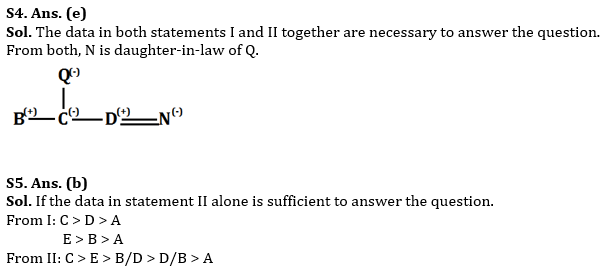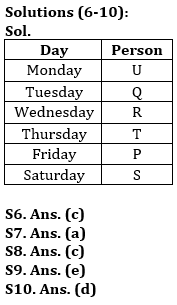Latest Banking jobs   »

# Reasoning Quiz For RBI Grade B Phase 1 2023-02nd June

Directions (1-5): Each of the questions below consists of a question and two statements numbered I and II given below it. You have to decide whether the data provided in the statements are sufficient to answer the question.
(a) If statements I alone is sufficient to answer the question, but statement II alone is not sufficient to answer the question.
(b) If statement II alone is sufficient to answer the question, but statement I alone is not sufficient to answer the question.
(c) If statement either I or II is sufficient to answer the question.
(d) If both the statements I and II taken together are not sufficient to answer the questions.
(e) If both the statements I and II taken together are sufficient to answer the questions.

Q1. What is Sonali’s position from the left end of a row?
I. There are 4 students between Gauri and Sonali. Bhavna is 6th to the right of Gauri.
II. Gini is 6th to the left of Sonali and is 2nd from the left end.

Q2. How many students are sitting between P and Q?
I. P is 5th to the right of R and 6th to the left of S. Q is 6th to the right of R.
II. In a row of 25 students, P is 5th from left end and Q is 20th from the right end.

Q3. Among G, H, I, J and K who among the following got third highest marks?
I. G has got 1 mark less than H and has got more marks than K.
II. I and J have got more marks than G.

Q4. How is N related to Q?
I. C is daughter of Q who is also the mother of D.
II. B is brother of C and N is the wife of D.

Q5. Who has got highest marks among A, B, C, D and E?
I. D has got greater marks than A but less than C. B has got greater marks than A but less than E.
II. E has got less marks than C but greater than B and D. A has got the lowest marks.

Directions (6-10): Study the information carefully and answer the questions given below.
Six persons P, Q, R, S, T and U are going on holiday on six different days of week (week starts from Monday to Saturday). All information is not necessarily in same order.
Not more than one person goes between Q and T who goes on Thursday. Two persons go between T and U. More than one person goes between Q and P. There is as many persons between U and R as many between R and P. Q does not go on Saturday.

Q6. How many persons go between Q and P?
(a) One
(b) Three
(c) Two
(d) Four
(e) None

Q7. Who among the following person goes just before P?
(a) T
(b) R
(c) Q
(d) R
(e) None of these

Q8. Who among the following person goes on Tuesday?
(a) U
(b) P
(c) Q
(d) R
(e) None of these

Q9. How many persons goes before U?
(a) Two
(b) One
(c) Four
(d) Five
(e) None

Q10. Which of the following combination is true?
(a) Monday-Q
(b) Tuesday-R
(c) Tuesday-U
(d) Friday-P
(e) None is true

Solutions

Solutions (1-5):
S1. Ans. (b)
Sol. The data in statement II alone is sufficient to answer the question.
From I: It is given that Gauri is 5th to Sonali but not given left or right
From II: Gini is 2nd from left end, and then Sonali is 6th to right of Gini, so Sonali is 8th from left end.

S2. Ans. (c)
Sol. If the data either in statement I alone or statement II alone are sufficient to answer the question.
From either statement, it says that there is no one between P and Q, or 0 students between P and Q.

S3. Ans. (d)
Sol. Both statements even together are not sufficient to answer.## FAQs

### How many sections are there in the RBI Grade B Phase 1 Exam?

There are 4 sections in the RBI Grade B Phase 1 Exam i.e. English Language, General Awareness, Quantitative Aptitude & Reasoning.

#### Congratulations!Union Budget 2023-24: Free PDF